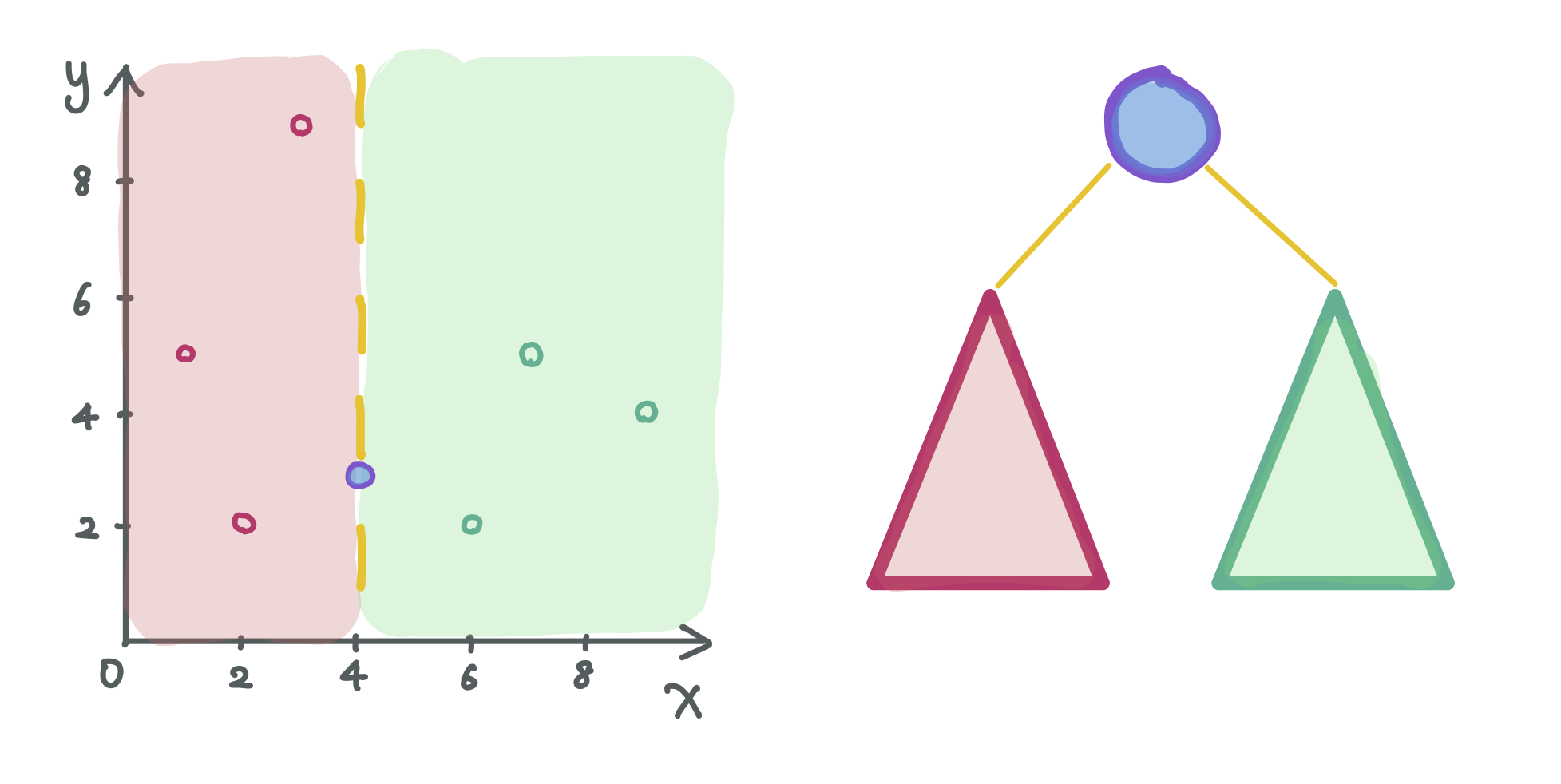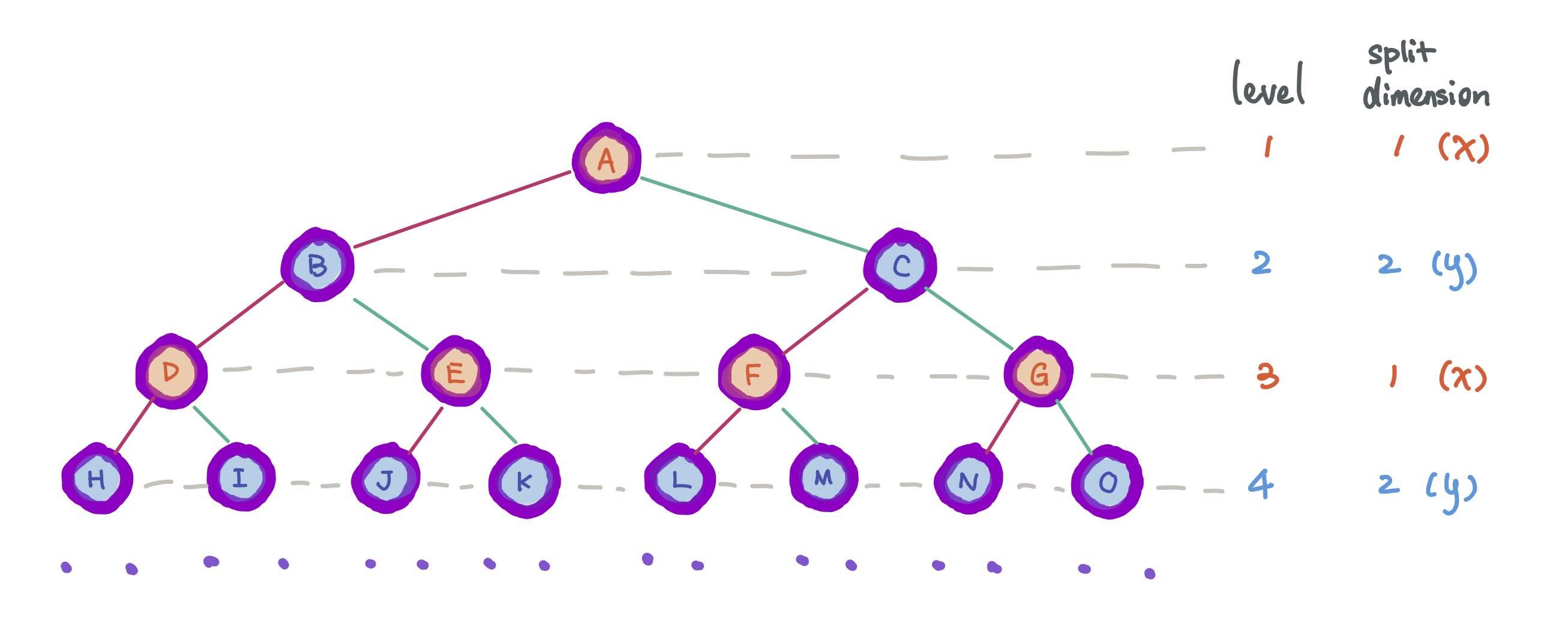Back to Resources

# k-d Trees

by Jenny Chen

## Overview

A k-d Tree is a binary search tree that organizes points in k dimensional space. Every node in a k-d Tree contains one point. Every parent node splits the space into two subspaces based on a certain dimension. It splits the space such that every node in its left subtree is in the left subspace, and every node in its right subtree is in the right subspace. The dimension that a node is splitting on depends on which level of the tree this node is in.The blue node is splitting on the first dimension (x axis). Every node has a smaller x coordinate belongs to its left subtree, and every node has a greater x coordinate belongs to its right subtree.

## Constructing a k-d Tree

In this course, we focus on constructing a balanced k-d Tree based on an array of points.

#### Alternate splitting dimension

As mentioned before, each node splits the space in a certain dimension. Conventionally, the nodes on the i th level split the space in the i th dimension. If i is greater than k, the dimension wraps back around to i mod k.This is an example of k-d Tree storing points in 2D. Each node on a odd level splits the x dimension, and each node on a even level splits the y dimension.

#### Find the median

In order to construct a balanced k-d Tree, each node should split the space such that there are an equal number of nodes in the left subspace as the right subspace. Therefore we need to pick the median among the nodes for the current dimension and make it the subroot.

#### Recurse on subtrees

After finding the subroot, put all nodes that are in the left subspace into one array, and the rest into another array. Repeat the process on the subarrays.